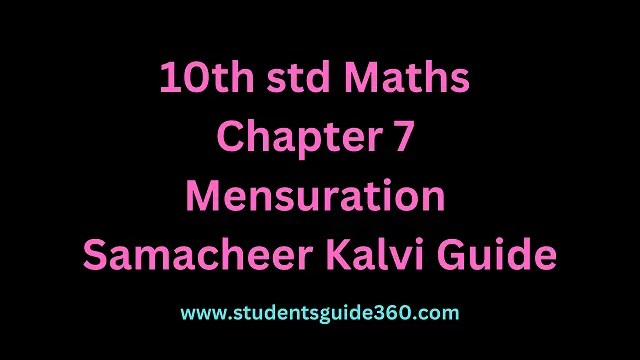# 10th Maths Chapter 7 Mensuration Exercise 7.1

10th Standard Maths Chapter 7 Mensuration Exercise 7.1 Guide. TN SSLC Samacheer Kalvi Guide Chapter 7 Exercise 7.1 Book Back Answers & Solutions. 10th All Subject Guide – Click Here. Class 1 to 12 All Subject Book Back Answers – Click Here.### 1. The radius and height of a cylinder are in the ratio 5:7 and its curved surface area is 5500 sq.cm. Find its radius and height.

Solution:
r = 5x
h = 7x
CSA of a cylinder = 2πrh∴ Radius = 5 × 5 = 25 cm
height = 7 × 5 = 35 cm

### 2. A solid iron cylinder has total surface area of 1848 sq.m. Its curved surface area is five – sixth of its total surface area. Find the radius and height of the iron cylinder.

The external radius and the length of a hollow
Solution:### 3. The external radius and the length of a hollow wooden log are 16 cm and 13 cm respectively. If its thickness is 4 cm then find its T.S.A.

Solution:
R = 16 cm
r = R – thickness
r = 12 cm
= 16 – 4 = 12 cm
h = 13 cm
Total surface area of hollow cylinder = 2π(R + r) (R – r + h) sq. units.∴ T.S.A = 2992 cm2

### 4. A right angled triangle PQR where ∠Q = 90° is rotated about QR and PQ. If QR = 16 cm and PR = 20 cm, compare the curved surface areas of the right circular cones so formed by the triangle.

Solution:
When it is rotated about PQ the C.S.A of the cone formed = πrl.1005.71 > 754.28
∴ CSA of the cone rotated about its PQ is larger than the CSA of the cone rotated about QR.

### 5. 4 persons live in a conical tent whose slant height is 19 cm. If each person require 22 cm2 of the floor area, then find the height of the tent.

Solution:
Base area of the cone = πr2 = sq units.### 6. A girl wishes to prepare birthday caps in the form of right circular cones for her birthday party, using a sheet of paper whose area is 5720 cm2, how many caps can be made with radius 5 cm and height 12 cm.

Solution:### 7. The ratio of the radii of two right circular cones of same height is 1 : 3. Find the ratio of their curved surface area when the height of each cone is 3 times the radius of the smaller cone.

Solution:### 8. The radius of a sphere increases by 25%. Find the percentage increase in its surface area.

Solution:
Surface area of sphere A = 4πr2
New radius = r’ = 1.25r,
[∵ r + 0.25r] (25% = 0.25)
New surface area = A’ = 4π(r’)2
= 4π(1.25 r)2### 9. The internal and external diameters of a hollow hemispherical vessel are 20 cm and 28 cm respectively. Find the cost to paint the vessel all over at ₹ 0.14 per cm2.

Solution:
External diameter D = 28 cm
Internal diameter d = 20 cm### 10. The frustum shaped outer portion of the table lamp has to be painted including the top part. Find the total cost of painting the lamp if the cost of painting 1 sq.cm is ₹ 2.Solution:
Here given that R = 12 m
r = 6 m
h = 8 m